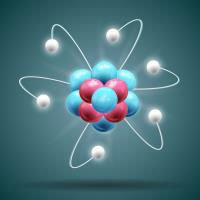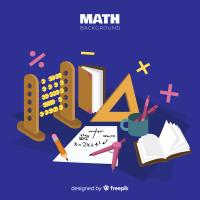Courses

# Notes | EduRev

## JEE: Notes | EduRev

The document Notes | EduRev is a part of the JEE Course NEET Revision Notes.
All you need of JEE at this link: JEE
• Frame of reference:- A system of coordinates whose axes can be suitably chosen is said to be a frame of reference.
• Inertial frame:- A frame of reference either at rest or moving with a uniform velocity (zero acceleration) is known as inertial frame.
• Non-inertial or accelerated frame:- It is a frame of reference which is either having a uniform linear acceleration or is being rotated with a uniform speed.
• Galilean transformation:-
(a) Transformation of position:-
x' = x – vt
y' = y
z' = z
t' = t
Inverse transformation,
x = x'+ vt '
y = y'
z = z'
t = t'
(b) Transformation of distance:-
l ' = l
Here, l = x2-x1 is the length of rod as observed in frame S.
Anything which remains unchanged when observed from the two Galilean frames of reference is known as Galilean invariant.
(c) Transformation of velocity:-
u'= u– v
Inverse transformation:- u = u'+ v
(d) Transformation of acceleration:-
a' = a
Thus, the acceleration of body, as observed by two observers siting in two inertial frames, is same. Hence, acceleration is said to be Galilean invariant.
• Law of conservation of momentum:-
It states that the total momentum of an isolated system (no external force) always remains constant.
In S frame:- m1u1 + m2u= m1v1 + m2v2
In S ' frame:- m1u'1 + m2 u'2  = m1 v'1  + m2 v'2
Thus, the law of conservation of momentum is valid in S ' also, indicating that the law is Galilean invariant.
• Ether and velocity of light:- c' = c ±v
• Postulates of special theory of relativity:-
(a) The laws of physical phenomenon are same when stated in terms of two systems of reference in uniform translator motion relative to each other.
(b) The velocity of light in vacuum is constant, independent not only of the direction of propagation but also of the relative velocity of the source and the observer.
• Lorentz transformation equations:-
x' = [x - vt]/√[1- v2/c2]
y'= y
z'= z
t' = [t – [(v/c 2) x]]/√[1- v2/c2]
• Lorentz inverse transformation equations:-
x= [x' + vt] /√[1- v2/c2]
y = y'
z= z'
t= [t' + [(v/c2) x']]/√[1- v2/c2]
• Length contraction:-
l = l0√[1- v2/c2]
So, l<l0
Here, l0 is the proper or original length in S frame and l is the relativistic length in S' frame.
• Time Dilation:-
??t = ??t0/√ [1-v2/c2]
So, ?t > ??t0
Here, ??t0 is the proper or original time in S frame and ?t is the relativistic time in S' frame.
?Thus, the time interval as observed by the moving observer appears to be lengthened.
• Frequency:- If f0 is the natural frequency of a process in frame S, then the frequency f as observed from S ' given by,
f = f0√[1- v2/c2]
ux' = [ux – v]/[1- [(v/c2)ux]]
uy' = [uy√[1- v2/c2]]/[1- [(v/c2)ux]]
uz' = [uz√[1- v2/c2]]/[1- [(v/c2)ux]]
• Relativistic variation of mass:-
m= m0/√[1-v2/c2]
• Rest mass energy:-
E0 = m0c2
• Total energy (Mass-Energy Equivalence):-
E= mc2 = [m0/√[1-v2/c2]] c2
• Kinetic energy:-
EK= E - E0 = (m - m0) c2
Here c is the speed of light, m0 is the rest mass and m is the relativistic mass.
• Relativistic momentum:-
p= mv
= [m0/√[1-v2/c2]] v
The document Notes | EduRev is a part of the JEE Course NEET Revision Notes.
All you need of JEE at this link: JEEUse Code STAYHOME200 and get INR 200 additional OFF Use Coupon Code
All Tests, Videos & Notes of JEE: JEE

### Up next### Top Courses for JEE## NEET Revision Notes

291 docs

### Up next### Top Courses for JEETrack your progress, build streaks, highlight & save important lessons and more!

,

,

,

,

,

,

,

,

,

,

,

,

,

,

,

,

,

,

,

,

,

;